# Handout 2: Different numbers of samples and different sample sizes

A downloadable version of this activity is available in the following format:

 1. Dataset  N= 100 Mean: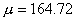Standard deviation: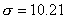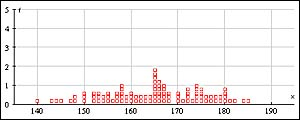2. 100 samples  n = 30 Mean: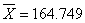Standard deviation:= 1.87734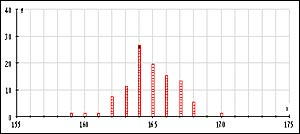3. 500 samples  n = 30 Mean:Standard deviation:= 1.87163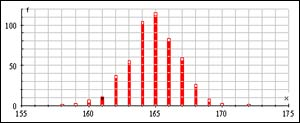4. 1,000 samples  n = 30 Mean: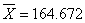Standard deviation:= 1.87355. 500 samples  n = 50 Mean: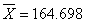Standard deviation:= 1.47276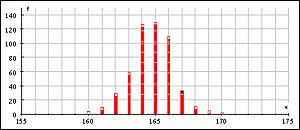6. 1,000 samples  n = 50 Mean: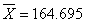Standard deviation:= 1.48305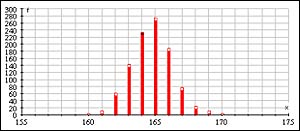This entry was posted in Teacher Resources. Bookmark the permalink.